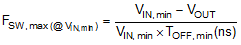ZHCSCY3E October   2014  – October 2018

PRODUCTION DATA.

1. 特性
2. 应用
3. 说明
1.     Device Images
4. 修订历史记录
5. Pin Configuration and Functions
6. Specifications
7. Detailed Description
1. 7.1 Overview
2. 7.2 Functional Block Diagram
3. 7.3 Feature Description
4. 7.4 Device Functional Modes
8. Application and Implementation
1. 8.1 Application Information
2. 8.2 Typical Applications
1. 8.2.1 LM5160 Synchronous Buck (10-V to 60-V Input, 5-V Output, 1.5-A Load)
2. 8.2.2 LM5160 Isolated Fly-Buck (18-V to 32-V Input, 12-V, 4.5-W Isolated Output)
3. 8.2.3 LM5160A Isolated Fly-Buck (18-V to 32-V Input, 12-V, 4.5-W Isolated Output)
3. 8.3 Do's and Don'ts
9. Power Supply Recommendations
10. 10Layout
11. 11器件和文档支持
1. 11.1 器件支持
1. 11.1.1 第三方产品免责声明
2. 11.1.2 开发支持
2. 11.2 文档支持
3. 11.3 相关链接
4. 11.4 接收文档更新通知
5. 11.5 社区资源
6. 11.6 商标
7. 11.7 静电放电警告
8. 11.8 术语表
12. 12机械、封装和可订购信息

• DNT|12

#### 8.2.1.2.3 Switching Frequency - RON

The duty cycle required to maintain output regulation at the minimum input voltage restricts the maximum switching frequency of the LM5160. The maximum value of the minimum forced off-time, TOFF,min, limits the duty cycle and therefore the switching frequency. Calculate the maximum frequency that avoids output dropout at minimum input voltage using Equation 10.

Equation 10.For this design example, the maximum frequency based on the minimum off-time limitation of TOFF,min (typical) = 170 ns is calculated as FSW,max(@VIN,min) = 2.9 MHz. This value is well above 1 MHz, the maximum possible operating frequency of the LM5160.

At maximum input voltage the maximum switching frequency of the LM5160 is restricted by the minimum on-time, TON,min which limits the minimum duty cycle of the converter. Calculate the maximum frequency at maximum input voltage using Equation 11.

Equation 11.Using Equation 11 and TON,min (typical) = 150 ns, the maximum achievable switching frequency is FSW,max(@VIN,min) = 514 kHz. Taking this value as the maximum possible switching frequency over the input voltage range for this application, choose a nominal switching frequency of FSW = 300 kHz for this design. The value of resistor RON sets the nominal switching frequency based on Equation 12.

Equation 12.For this particular application with FSW = 300 kHz, RON calculates to be 167 kΩ. Selecting a standard value for R3 (RON) = 169 kΩ (±1%) results in a nominal frequency of 296 kHz. The resistor value may need to adjusted further in order to achieve the required switching frequency as the switching frequency in COT converters varies slightly (±10%) with input voltage and/or output current. Operation at a lower nominal switching frequency results in higher efficiency but increases the inductor and capacitor values leading to a larger total solution size.